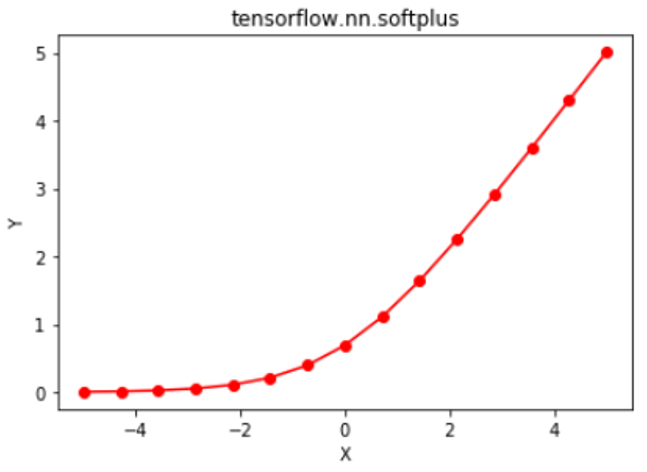# Python | Tensorflow nn.softplus()

Tensorflow is an open-source machine learning library developed by Google. One of its applications is to develop deep neural networks.

The module `tensorflow.nn` provides support for many basic neural network operations.

An activation function is a function which is applied to the output of a neural network layer, which is then passed as the input to the next layer. Activation functions are an essential part of neural networks as they provide non-linearity, without which the neural network reduces to a mere logistic regression model. One of the many activation functions is the Softplus function which is defined as.

Traditional activation functions such as sigmoid and hyperbolic tangent have lower and upper bounds, whereas the softplus function outputs in the range (0, ∞). The derivative of the softplus function comes out to be, which is the sigmoid function. The softplus function is quite similar to the Rectified Liner Unit (ReLU) function, with the main difference being softplus function’ differentiability at the x = 0. The research paper “Improving deep neural networks using softplus units” by Zheng et al. (2015) suggests that softplus provides more stabilization and performance to deep neural networks than ReLU function. However, ReLU is generally preferred because of the ease in calculating it and its derivative. Calculation of activation function and its derivative is a frequent operation in neural networks, and ReLU provides faster forward and backward propagation when compared with softplus function.

The function `nn.softplus()` [alias `math.softplus`] provides support for softplus in Tensorflow.

Syntax: tf.nn.softplus(features, name=None) or tf.math.softplus(features, name=None)

Parameters:
features: A tensor of any of the following types: float32, float64, int32, uint8, int16, int8, int64, bfloat16, uint16, half, uint32, uint64.
name (optional): The name for the operation.

Return type: A tensor with the same type as that of features.

Code #1:

 `# Importing the Tensorflow library ` `import` `tensorflow as tf ` ` `  `# A constant vector of size 6 ` `a ``=` `tf.constant([``1.0``, ``-``0.5``, ``3.4``, ``-``2.1``, ``0.0``, ``-``6.5``], dtype ``=` `tf.float32) ` ` `  `# Applying the softplus function and ` `# storing the result in 'b' ` `b ``=` `tf.nn.softplus(a, name ``=``'softplus'``) ` ` `  `# Initiating a Tensorflow session ` `with tf.Session() as sess: ` `    ``print``(``'Input type:'``, a) ` `    ``print``(``'Input:'``, sess.run(a)) ` `    ``print``(``'Return type:'``, b) ` `    ``print``(``'Output:'``, sess.run(b)) `

Output:

```Input type: Tensor("Const:0", shape=(6, ), dtype=float32)
Input: [ 1.        -0.5        3.4000001 -2.0999999  0.        -6.5      ]
Return type: Tensor("softplus:0", shape=(6, ), dtype=float32)
Output: [  1.31326163e+00   4.74076986e-01   3.43282866e+00   1.15519524e-01
6.93147182e-01   1.50233845e-03]
```

Code #2: Visualization

 `# Importing the Tensorflow library ` `import` `tensorflow as tf ` ` `  `# Importing the NumPy library ` `import` `numpy as np ` ` `  `# Importing the matplotlib.pylot function ` `import` `matplotlib.pyplot as plt ` ` `  `# A vector of size 15 with values from -5 to 5 ` `a ``=` `np.linspace(``-``5``, ``5``, ``15``) ` ` `  `# Applying the softplus function and ` `# storing the result in 'b' ` `b ``=` `tf.nn.softplus(a, name ``=``'softplus'``) ` ` `  `# Initiating a Tensorflow session ` `with tf.Session() as sess: ` `    ``print``(``'Input:'``, a) ` `    ``print``(``'Output:'``, sess.run(b)) ` `    ``plt.plot(a, sess.run(b), color ``=` `'red'``, marker ``=` `"o"``)  ` `    ``plt.title(``"tensorflow.nn.softplus"``)  ` `    ``plt.xlabel(``"X"``)  ` `    ``plt.ylabel(``"Y"``)  ` ` `  `    ``plt.show() `

Output:

```Input: [-5.         -4.28571429 -3.57142857 -2.85714286 -2.14285714 -1.42857143
-0.71428571  0.          0.71428571  1.42857143  2.14285714  2.85714286
3.57142857  4.28571429  5.        ]
Output: [ 0.00671535  0.01366993  0.02772767  0.05584391  0.11093221  0.21482992
0.39846846  0.69314718  1.11275418  1.64340135  2.25378936  2.91298677
3.59915624  4.29938421  5.00671535]
```Attention geek! Strengthen your foundations with the Python Programming Foundation Course and learn the basics.

To begin with, your interview preparations Enhance your Data Structures concepts with the Python DS Course.

My Personal Notes arrow_drop_upCheck out this Author's contributed articles.

If you like GeeksforGeeks and would like to contribute, you can also write an article using contribute.geeksforgeeks.org or mail your article to contribute@geeksforgeeks.org. See your article appearing on the GeeksforGeeks main page and help other Geeks.

Please Improve this article if you find anything incorrect by clicking on the "Improve Article" button below.

Improved By : sanskar27jain

Article Tags :

Be the First to upvote.

Please write to us at contribute@geeksforgeeks.org to report any issue with the above content.